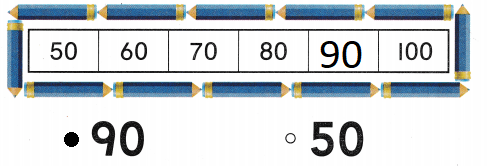# Texas Go Math Kindergarten Lesson 16.4 Answer Key Count from Any Number

Refer to our Texas Go Math Kindergarten Answer Key Pdf to score good marks in the exams. Test yourself by practicing the problems from Texas Go Math Kindergarten Lesson 16.4 Answer Key Count from Any Number.

## Texas Go Math Kindergarten Lesson 16.4 Answer Key Count from Any Number

Essential Question
How can you solve problems using logical reasoning?

Unlock the ProblemA number greater than 38
The number after 38 is 39
Explanation: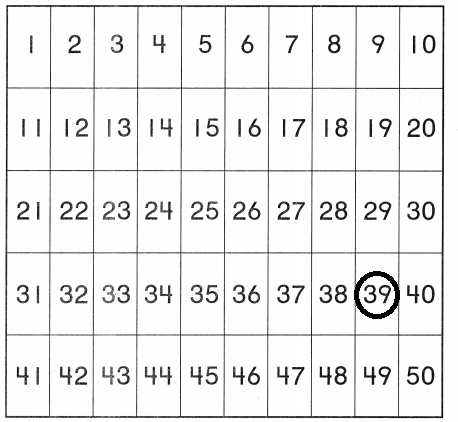Directions
Listen to each number riddle. Use the fifty chart and the clues to solve each riddle. Count from the secret number to 50.

Try Another Problem

Question 1.Explanation:
The given secret number is 56
so, circled the number 56 and
started counting from 56.

Directions
1. Listen to each number riddle, Use the hundred chart and the clues to solve each riddle. Count from the secret number to 100.

Share and Show

Question 2.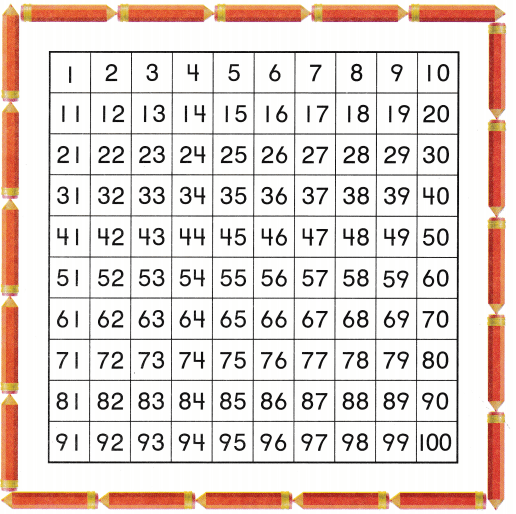Explanation:
The given secret number is 87
so, circled the number 87 and
started counting from 87.

Directions
2. Listen to each number riddle. Use the hundred chart and the clues to solve each riddle. Count from the secret number to 100.

Home Activity

• Give your child a hundred chart, Say a number riddle. For example, I am greater than 40. I am less than 12. What number am I? Have your child circle the number on the chart.

Question 3.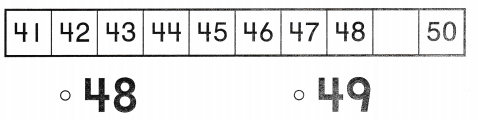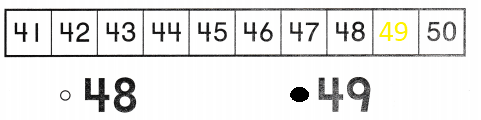Explanation:
The given series of numbers are from 41 to 50
The number after 48 is 49
so, the missing number is 50

Question 4.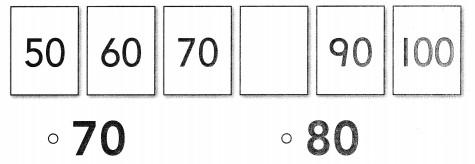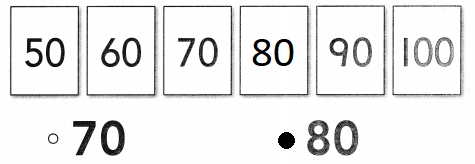Explanation:
The given pattern is count by 10’s
so, after 70 the missing number is 80.

Question 5.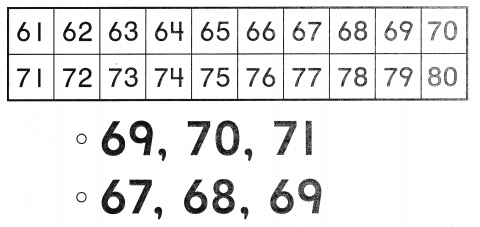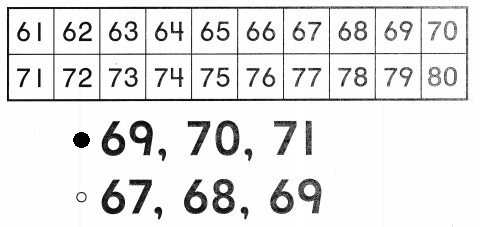Explanation:
The three numbers comes after 68 are
69 , 70, 71 so, marked these three numbers.

Directions
Choose the correct answer. 3. Point to each number as you count. What is the missing number? 11. Count by tens. What is the missing number? 5. Which three numbers come after 68?

### Texas Go Math Kindergarten Lesson 16.4 Homework and Practice

Question 1.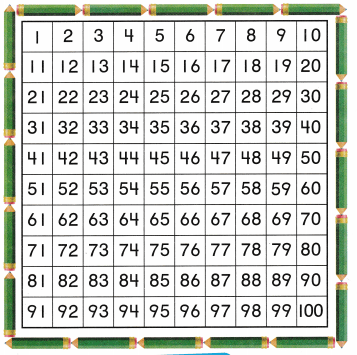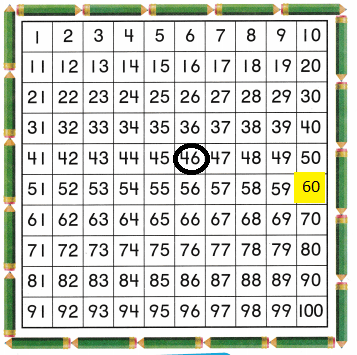Explanation:
I am between 41 and 50. I am one more than 45.
so, the number is 46 and circled the number 46.
I am greater than 50. I am less than 70.
so, the counting is skip by 10’s
The number after 50 is 60
so, colored the box 60

Directions
1. Solve the number riddles.
Riddle I: I am between 41 and 50. I am one more than 45. What number am I? Circle the number. Count from this number to 100. Riddle 2: You say my number when you count by tens. I am greater than 50. I am less than 70. What number am I? Color the box with my number red. Count from this number to 100.

Texas Test Prep

Lesson Check

Question 2.Explanation:
In the given series of numbers the missing number is 16
so, marked the number 16.

Question 3.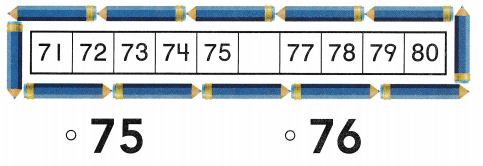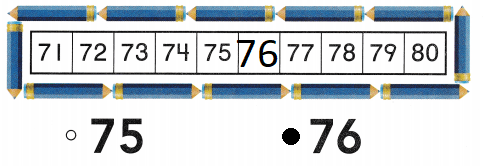Explanation:
The number after 75 is 76
so, the missing number is 76
marked the number 76.

Question 4.